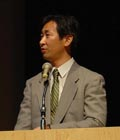# Takaaki KajitaTakaaki Kajita

### Research accomplishments:

I have been working in Kamiokande and Super-Kamiokande experiments. In particular, I have been studying atmospheric neutrinos and neutrino oscillations. In 1988, we discovered the atmospheric muon-neutrino deficit (this was called atmospheric neutrino anomaly), which was confirmed to be due to neutrino oscillations 10 years later. In this study we showed that the nm / ne ratio observed in Kamiokande was only about 60% of the predicted ratio. Subsequently, in 1994, we discovered that the atmospheric muon-neutrino deficit depends on the zenith-angle or equivalently on the neutrino flight length through the study of multi-GeV atmospheric neutrino events observed in Kamiokande, which was the another indication for the neutrino oscillations.

In 1996 the Super-Kamiokande experiment started. I have been leading the studies of atmospheric neutrinos in this experiment. In 1998, by the study of the high statistics data from Super-Kamiokande, we concluded that the observed atmospheric muon-neutrino deficit was due to neutrino oscillations. The result was presented at the Neutrino 98 conference. We have been studying neutrino oscillations further. Recent major accomplishments are the confirmation of nmànt oscillations rather than oscillations to sterile neutrinos in 2000, the observation of sinusoidal muon-neutrino disappearance as predicted by the neutrino oscillation formula in 2004, and the first indication of appearance of tau-neutrinos which are generated by neutrino oscillations in 2006. In these studies we have established the standard neutrino oscillation generated by neutrino masses and mixing-angles.

### Current research objectives:

1. So far the atmospheric neutrino data are explained well by two flavor nmànt oscillations. However, at some level, three flavor oscillation effects should be visible. Especially, the effect of the third and yet-unknown mixing angle, q13, might be visible as an excess of multi-GeV, upward-going ne events. Also, the effect of the solar oscillation terms (driven by Dm122) should be observed in the sub-GeV upward-going neutrino events due to the very long flight length of the neutrinos. Therefore, we are studying the 3 flavor structure of neutrino oscillations using atmospheric neutrinos.
2. If q13 is very small (sin22q13 < 0.05), current atmospheric neutrino experiments might not be sensitive to q13. Therefore, we are also working in a long baseline neutrino oscillation experiment, T2K. This experiment will start in 2009, and will be the most sensitive experiment to a small q13. Especially, we are working for the intermediate detector to get the best possible discovery potential of this experiment for non-zero q13. We are also working in the Super-Kamiokande analysis of the T2K neutrino events.
3. If a non-zero q13 is discovered, it will become possible to study the CP violation in the neutrino sector, which is believed to be very important related to the baryon asymmetry of the Universe. However, it is known that a long baseline neutrino experiment with a very high intensity neutrino beam together with a very large detector is necessary. For this purpose, we are carrying out the R&D studies for the future Mega-ton-class water Cherenkov detector. Furthermore, preliminary studies showed that a neutrino oscillation experiment with the JPARC neutrino beam and large detectors at Kamioka and Korea will expand the sensitivity of the experiment to the mass hierarchy measurement and the possible determination of the q23 angle without any ambiguity. Therefore, we are studying the physics potential of these future neutrino oscillation experiments.